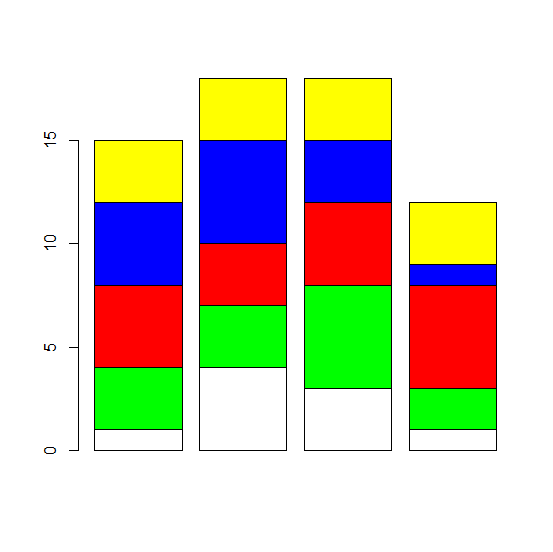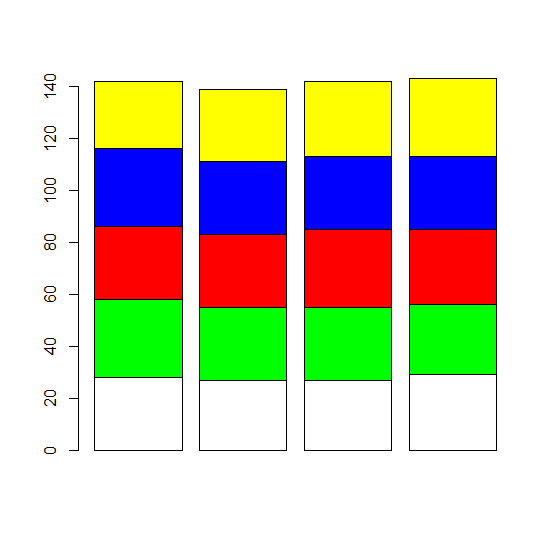# How to create stacked barplot using barplot function with each bar having unique color in R?

In a bar plot, each bar represents one category of a single categorical variable but in a stacked bar plot, the bars represent same categorical variable but each divided into sub-categories. If we want to have similar distribution of colors in each bar then col argument with barplot function can be used.

## Example1

Live Demo

M1<−matrix(sample(c(1:5),20,replace=TRUE),ncol=4)
M1

## Output

   [,1] [,2] [,3] [,4]
[1,] 4    3    5    3
[2,] 4    2    1    4
[3,] 5    5    2    3
[4,] 3    2    2    4
[5,] 1    5    2    5

## Example

barplot(M1,col=rep(c("white","green","red","blue","yellow"),5))

## Output## Example2

Live Demo

M2<−matrix(sample(c(26:30),20,replace=TRUE),ncol=4)
M2
[,1] [,2] [,3] [,4]
[1,] 28 27 27 29
[2,] 30 28 28 27
[3,] 28 28 30 29
[4,] 30 28 28 28
[5,] 26 28 29 30

## Example

barplot(M2,col=rep(c("white","green","red","blue","yellow"),5))

## Output## Example

M3<−matrix(rpois(20,5),ncol=4)
M3

## Output

[,1] [,2] [,3] [,4]
[1,] 6 8 4 5
[2,] 8 3 7 2
[3,] 5 4 4 2
[4,] 5 3 5 2
[5,] 6 5 2 3

## Example

barplot(M3,col=rep(c("white","green","red","blue","yellow"),5))

## OutputUpdated on: 07-Nov-2020

243 Views